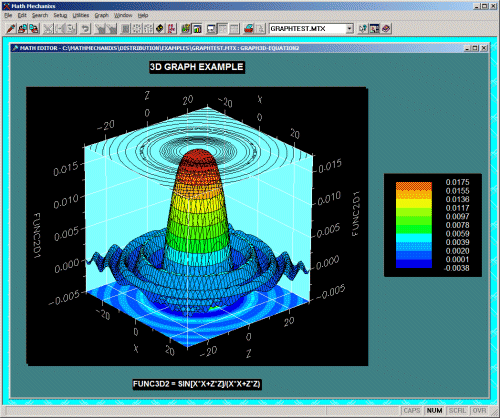# Math Mechanixs

Developer:
Version:
Windows 2000 Windows XP - English

Mathematics is a real challenge for most people. Mechanixs Math has been designed to assist in the analysis of a mathematical problem. It also serves as a calculator, a program with multiple functions.# Key Features

• Resolution: with Mechanixs Math’s data analysis tools, it is possible to solve mathematical problems of all kinds, for example, logarithmic, trigonometric, statistical or logical.
• Analysis: mathematical analysis performed using this software are diverse, ranging from simple reasoning to complex statistical analyzes. This software does not stop the calculations but performs graphical analyzes.
• Database: this program has a database with many predefined functions, features Solver, 2D and 3D graphics, etc. The elements of the library can solve all transactions with ease and convenience.
• Calculator: however, it is possible to perform simple calculations with Mechanixs Math. It has a calculator including basic operations such as addition, subtraction, multiplication, addition.

# System requirements

• Operating Systems: Windows 98, Windows Me, Windows 2000, Windows XP.
• Processor: Pentium 233MHz.

# Pros

• Mechanixs Math is an award-winning tool.
• Its interface is intuitive and ergonomically designed for easy handling.

# Cons

• This tool is not available in other languages.
• After thirty days of use, you have to pay for a license.
Related
Alternative spelling: mathmechsetup-1.5.0.3.exe, mathmechsetup.exe